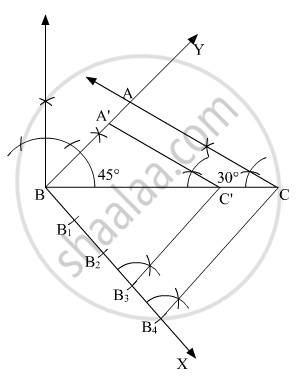# Construct a Triangle Abc with Sides Bc = 7 Cm, ∠B = 45° and ∠A = 105°. Then Construct a Triangle Whose Sides Are 3/4 Times the Corresponding Sides of ∆Abc. - Mathematics

Construct a triangle ABC with sides BC = 7 cm, ∠B = 45° and ∠A = 105°. Then construct a triangle whose sides are 3/4 times the corresponding sides of ∆ABC.

#### Solution

Steps of construction:

1. Draw line BC = 7 cm

2. At B, construct ∠CBY = 45° and at C, construct 180° −(105° + 45°) = 30°.

3. Mark the point of intersection of ∠B = 45° and ∠C = 30° as A. Thus, ∆ABC is obtained.

4. Draw any ray BX making an acute angle with BC on the side opposite to the vertex A.

5. Locate 4 (3 < 4) points B1, B2, B3 and B4 on BX such that  BB1 = B1B2 = B2B3 = B3B4.

6. Join B4C and draw a line through B3 parallel to B4C to intersect BC at C'.

7. Draw a line through C′ parallel to the line CA to intersect BA at A′

Then, ΔA′BC′ is the required triangle similar to the ΔABCConcept: Application of Pythagoras Theorem in Acute Angle and Obtuse Angle
Is there an error in this question or solution?